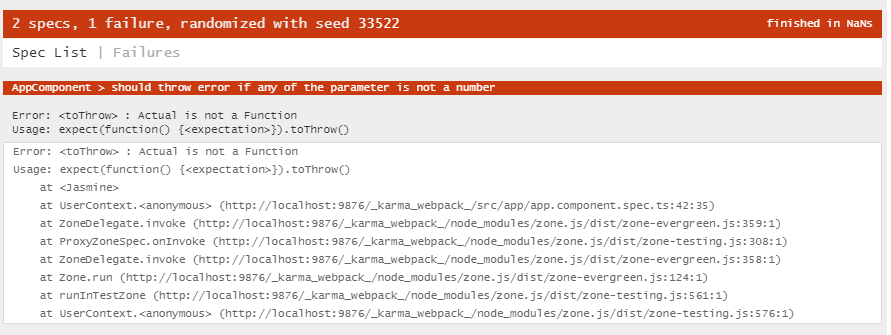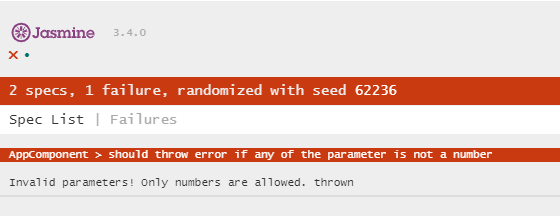## Introduction

This is the second tutorial of the Unit test in Angular series where the goal is to make unit tests in Angular simple and easy to understand for everyone. In the last tutorial, Introduction to Unit Test in Angular, we covered the basic concepts and terminologies in unit tests. In this tutorial, we will introduce the common pattern to follow while writing a test case then introduce some common Jasmine functions that we can use in our unit tests.

## The Triple A Pattern

When writing a test case, disregarding it’s a unit test or a functional test, the very commonly followed pattern is the Arrange – Act – Assert pattern, or also known as the Triple A Pattern.

Arrange – Act – Assert stands for:

Arrange – Prepare the test environment, such as test data or the initial state of each variable before the test begins. In Backend testing this may refer to data in the Database, but in the Frontend testing, this could be data from APIs, or simply put variables in the class.

Act – The action that we will perform during this test case. In Frontend testing, actions could include interaction with a DOM element, invoking a function or making an API call.

Assert – The expected result or outcome after the action was made. This is where tests really happen and where we can test if our programs have met our expectations.

This pattern is so popular because while you are following the pattern to prepare a test case, it forces you to think ahead of time and ask yourself the following questions:

1. What is the purpose of this test case?
2. What is the main target that will be tested in this test case?
3. In order to test this target, what initial states should be prepared and what variables or functions will be involved in this test?
4. What is the expected result and how can one define if the test case can be considered as passed or failed?

If your test case can answer these questions, then congratulations you are on the right track. In addition, it is important to keep in mind that a test case should only be built to test one thing. In other words, if you have multiple things you want to test, consider separating them into different test cases. This will help you debug and identify what is wrong with your code if in the future there are failing tests due to new features added to your program.

## Matchers

Matchers are functions provided by the Jasmine framework to test if something matches our expectations. In Frontend testings, these Matcher functions start with the keyword expect.

For example:

In the test case above, we prepare some initial variables (a and b), perform some action on them and save the result to the variable c, then finally compare the result on line 45 with the toBeLessThan matcher. Of course usually line 42 would be in the ts file in the function you want to test against, so line 42 can usually be replaced by invoking a function from the component.

And here the test result:

There are many useful matchers

``````expect().toBeTruthy()
expect().toBeFalsy()
expect().toBeNull()
expect().toEqual()
expect().toContain()
expect().toHaveBeenCalled()``````

Here’s a Jasmine cheatsheet that I used to refer a lot when I started to write Angular tests:
https://devhints.io/jasmine

## Our first test case

Let’s try to write some tests using what we have learned in this tutorial.

Suppose we would like to test against a simple function – sum. The function is expected to take in two variables and return the sum of it. We would like to write some tests against this function, and gradually improve the function if we found issues while writing the tests.

The start point of our function:

For our first test case, the most intuitive thing to test is of course the output of the function, so let’s try to write a test case for that.

For the Arrange state, we prepare two variables that we will be using during this test. (line 26, 27). Then we call the sum function from the test environment by accessing it through the component instance in the Act state (line 30).

Note. all variables or functions can be accessed through the component instance.

Finally, we make our assertion in the Assert state to check against the result.

While you could end your test case here, it’s always a good practice to test your functions with various situations. For instance, what if one of the parameters is not provided to the function? Instead of crashing the program, we would like to throw an error and let the user know they need to provide additional parameters to the function.

For this purpose, we developed the following test case:

Since we are writing programs in TypeScript, it checks if all parameters are passed into the function and mark errors for us if missing. Therefore, instead of checking against missing parameters, we change one parameter to be null during the Arrange state (line 38, 39). We expect our function to throw an error if any of the parameters is not a number.

Notice that this time Act and Assert are combined together into a single line of code. This is because we expect the function to throw an error when we call it, so if we save the output to a variable like we did in the first test case, the program will never reach the Assert statement.

Without updating the function, we get the following error from Jasmine.Initial attempt of verifying function inputs

Well, this is expected because we haven’t updated our function yet. Let’s update the function, so the test case will pass.

We use the native typeof function from JavaScript to verify if both parameters are numbers before we continue.

After we updated the function, we got the following output from Jasmine:Verify Input test case failed again

We threw an error from our function, but the test was still failing. Why? Whenever we need to test if errors are thrown from a function, we must always wrap our function call in an anonymous function.